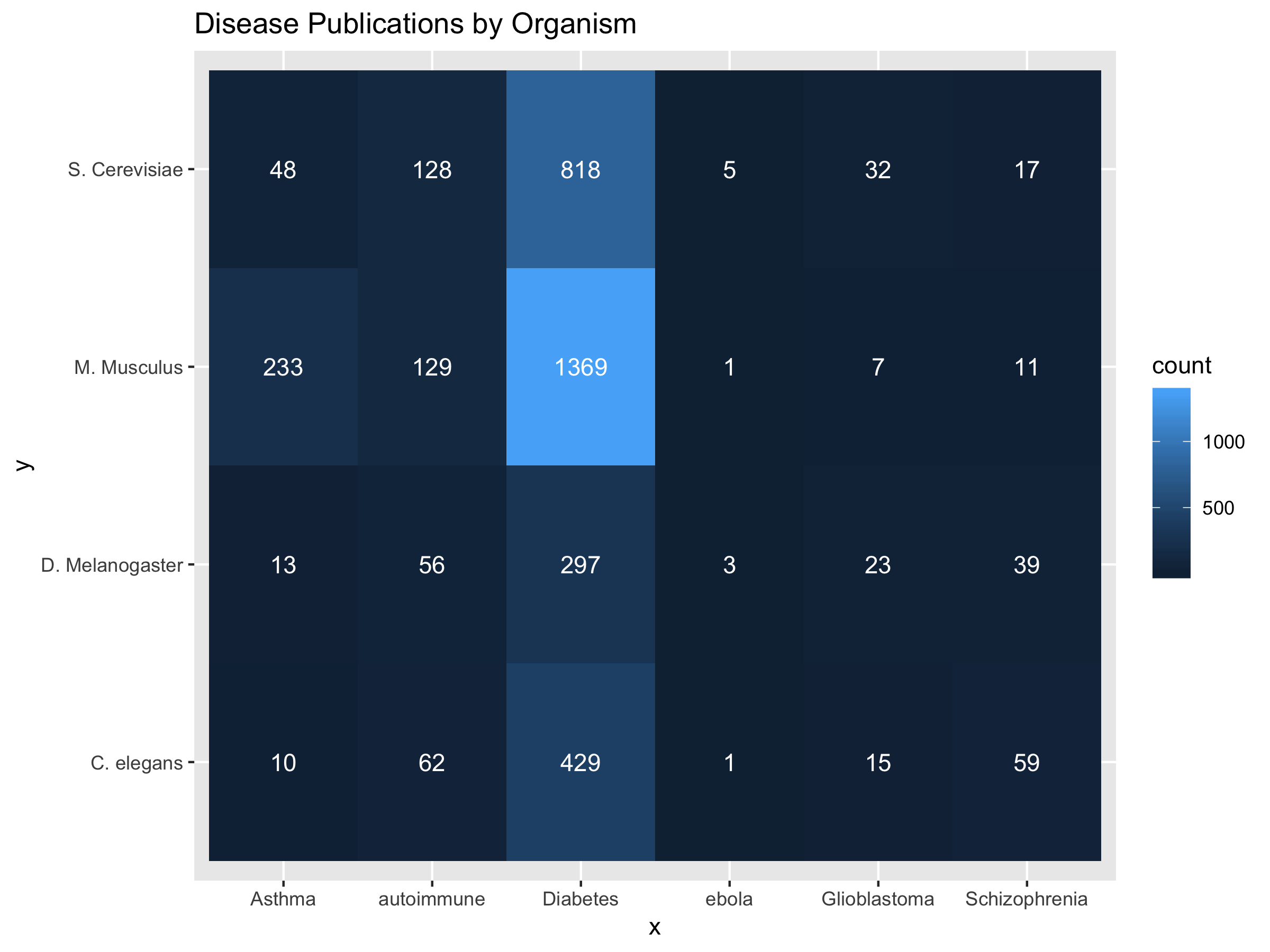You can look for interesting associations between sets of search terms on PubMed by comparing how often two terms co-occur. The code below returns the number of publications where both terms are mentioned, acting as a rough estimate for how associated they are (at least, in the scholarly world).

In the example below, I show the results from organisms x diseases/disease-associated terms which is an imperfect look at how various terms estimate of how much each disease is studied in a given organism. Of course, this should all be taken with a (big) grain of salt because these organisms and diseases have many synonyms or related terms (e.g. M. Musculus is often referred to as Mouse in the literature). Additionally, the result count is based off of whether or not the terms were found together within the title and abstract of the literature only – and not the body of the text in many cases.

``````# install.packages("RISmed", "ggplot2")
library(RISmed)
library(ggplot2)

# Given two lists of terms, lets see how 'hot' they are together
set1 <- c("ebola","autoimmune","Diabetes","Glioblastoma","Asthma","Schizophrenia")
set2 <- c("C. elegans","D. Melanogaster", "M. Musculus","S. Cerevisiae")

# Generate all possible pairs
pairs <- expand.grid(set1, set2, stringsAsFactors=F)

# Search pubmed for each pair, and return the number of search results.
results <- lapply(1:nrow(pairs),  function(x) {
query <- sprintf("%s %s", pairs[x,]\$Var1, pairs[x,]\$Var2)
print(query)
result <- EUtilsSummary(query, type='esearch', db='pubmed')
c(q1=pairs[x,]\$Var1, q2=pairs[x,]\$Var2, count=QueryCount(result))
})

# Do some data formatting on the results.
results <- as.data.frame(do.call("rbind", results), stringsAsFactors=F)
# Turn the number of search results into numeric form.
results\$count <- as.numeric(results\$count)

# Plot the results using geom_tile
ggplot(results) +
geom_tile(aes(x=q1, y=q2, fill=count)) +
geom_text(aes(x=q1, y=q2, label=count), color = "white") +
labs(title="Disease Publications by Organism", x="x", y="y")

ggsave("~/Desktop/pairwise_search.png", width = 8, height = 6)``````